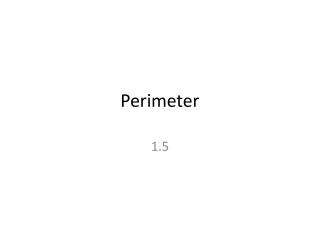DownloadDownload PresentationPerimeter

# Perimeter

Télécharger la présentation## Perimeter

- - - - - - - - - - - - - - - - - - - - - - - - - - - E N D - - - - - - - - - - - - - - - - - - - - - - - - - - -
##### Presentation Transcript

1. Perimeter 1.5

2. Perimeter • The outside measurement of a figure • Think of a fence

3. To find perimeter • Add up all the side lengths

4. Triangle • P= a+b+c

5. Rectangle • P=2 (length) +2 (width)

6. Square • P= 4 side length

7. Irregular figure • Try to divide it up to figures you know • Rectangle • Square • Triangle Add up all the outside figures.

8. Worksheet

9. Homework • Finish homework